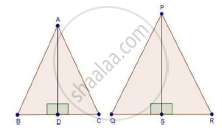# The Areas of Two Similar Triangles Are 25 Cm2 and 36 Cm2 Respectively. If the Altitude of the First Triangle is 2.4 Cm, Find the Corresponding Altitude of the Other. - Mathematics

The areas of two similar triangles are 25 cm2 and 36 cm2 respectively. If the altitude of the first triangle is 2.4 cm, find the corresponding altitude of the other.

#### SolutionWe have,

ΔABC ~ ΔPQR

Area (ΔABC) = 25 cm2

Area (ΔPQR) = 36 cm2

And AD and PS are the altitudes

To find: PS

Proof: Since, ΔABC ~ ΔPQR

Then, by area of similar triangle theorem

("Area"(triangleABC))/("Area"(trianglePQR))="AB"^2/"PQ"^2

rArr25/36="AB"^2/"PQ"^2

rArr5/6="AB"/"PQ"                 .........(i)

In ΔABD and ΔPQS

∠B = ∠Q [Δ ABC ~ ΔPQR]

Then, ΔABD ~ ΔPQS [By AA similarity]

therefore"AB"/"PS"="AD"/"PS"           ......(ii)   [Corresponding parts of similar Δ are proportional]

Compare (i) and (ii)

"AD"/"PS"=5/6

rArr2.4/"PS"=5/6

"PS"=(2.4xx6)/5=2.88 cm

Concept: Areas of Similar Triangles
Is there an error in this question or solution?

#### APPEARS IN

RD Sharma Class 10 Maths
Chapter 7 Triangles
Exercise 7.6 | Q 5 | Page 95